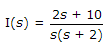# Electronics and Communication Engineering - Signals and Systems

Exercise : Signals and Systems - Section 4
1.
If f(t) is in volts, then F(jω) is in
volts
volt seconds
volts/sec
volt-sec2
Explanation:

Since we integrate with respect to time to F(jω), we get volt-secs.

2.
A voltage V(t) which is a Gaussian ergodic random process with a mean of zero and a variance of 4 volt2 is measured by a true rms meter. The reading will be
0
4
2
16
Explanation:

Rms Uvalue =4.

3.

Assertion (A): If, the final value of i(t) = 10

Reason (R): If, the initial value i(t) = 2

Both A and R are correct and R is correct explanation of A
Both A and R are correct but R is not correct explanation of A
A is true, R is false
A is false, R is true
Explanation:

Final value = 5. Hence A is wrong, initial value is 2. Hence R is correct.

4.
An ac circuit has an impedance of (3 + j6) ohm for fundamental. The impedance for fifth harmonic is
(15 + j 30) Ω
(15 + j 6) Ω
(3 + j 30) Ω
(3 + j 1.2) Ω
Explanation:

R is independent of w and XL ∝ ω.

5.
Which of following is recursive system?
y(n - 1)
y(n + 1)
y(n)
y(n) + y(n + 1)